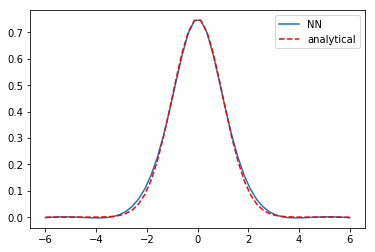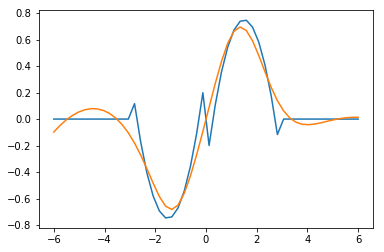## Solving an eigenvalue differential equation with a neural network

| categories: | tags: | View Comments

## Table of Contents

The 1D harmonic oscillator is described here. It is a boundary value differential equation with eigenvalues. If we let let ω=1, m=1, and units where ℏ=1. then, the governing differential equation becomes:

$$-0.5 \frac{d^2\psi(x)}{dx^2} + (0.5 x^2 - E) \psi(x) = 0$$

with boundary conditions: $$\psi(-\infty) = \psi(\infty) = 0$$

We can further stipulate that the probability of finding the particle over this domain is equal to one: $$\int_{-\infty}^{\infty} \psi^2(x) dx = 1$$. In this set of equations, $$E$$ is an eigenvalue, which means there are only non-trivial solutions for certain values of $$E$$.

Our goal is to solve this equation using a neural network to represent the wave function. This is a different problem than the one here or here because of the eigenvalue. This is an additional adjustable parameter we have to find. Also, we have the normalization constraint to consider, which we did not consider before.

## 1 The neural network setup

Here we setup the neural network and its derivatives. This is the same as we did before.

import autograd.numpy as np
from autograd import grad, elementwise_grad
import autograd.numpy.random as npr
from autograd.misc.optimizers import adam

def init_random_params(scale, layer_sizes, rs=npr.RandomState(42)):
"""Build a list of (weights, biases) tuples, one for each layer."""
return [(rs.randn(insize, outsize) * scale,   # weight matrix
rs.randn(outsize) * scale)           # bias vector
for insize, outsize in zip(layer_sizes[:-1], layer_sizes[1:])]

def swish(x):
"see https://arxiv.org/pdf/1710.05941.pdf"
return x / (1.0 + np.exp(-x))

def psi(nnparams, inputs):
"Neural network wavefunction"
for W, b in nnparams:
outputs = np.dot(inputs, W) + b
inputs = swish(outputs)
return outputs

psip = elementwise_grad(psi, 1) # dpsi/dx
psipp = elementwise_grad(psip, 1) # d^2psi/dx^2


## 2 The objective function

The important function we need is the objective function. This function codes the Schrödinger equation, the boundary conditions, and the normalization as a cost function that we will later seek to minimize. Ideally, at the solution the objective function will be zero. We can't put infinity into our objective function, but it turns out that x = ± 6 is practically infinity in this case, so we approximate the boundary conditions there.

Another note is the numerical integration by the trapezoid rule. I use a vectorized version of this because autograd doesn't have a trapz derivative and I didn't feel like figuring one out.

We define the params to vary here as a dictionary containing neural network weights and biases, and the value of the eigenvalue.

# Here is our initial guess of params:
nnparams = init_random_params(0.1, layer_sizes=[1, 8, 1])

params = {'nn': nnparams, 'E': 0.4}

x = np.linspace(-6, 6, 200)[:, None]

def objective(params, step):
nnparams = params['nn']
E = params['E']
# This is Schrodinger's eqn
zeq = -0.5 * psipp(nnparams, x) + (0.5 * x**2 - E) * psi(nnparams, x)
bc0 = psi(nnparams, -6.0) # This approximates -infinity
bc1 = psi(nnparams, 6.0)  # This approximates +infinity
y2 = psi(nnparams, x)**2
# This is a numerical trapezoid integration
prob = np.sum((y2[1:] + y2[0:-1]) / 2 * (x[1:] - x[0:-1]))
return np.mean(zeq**2) + bc0**2 + bc1**2 + (1.0 - prob)**2

# This gives us feedback from the optimizer
def callback(params, step, g):
if step % 1000 == 0:
print("Iteration {0:3d} objective {1}".format(step,
objective(params, step)))


## 3 The minimization

Now, we just let an optimizer minimize the objective function for us. Note, I ran this next block more than once, as the objective continued to decrease. I ran this one at least two times, and the loss was still decreasing slowly.

params = adam(grad(objective), params,
step_size=0.001, num_iters=5001, callback=callback)

print(params['E'])

Iteration   0 objective [[ 0.00330204]]
Iteration 1000 objective [[ 0.00246459]]
Iteration 2000 objective [[ 0.00169862]]
Iteration 3000 objective [[ 0.00131453]]
Iteration 4000 objective [[ 0.00113132]]
Iteration 5000 objective [[ 0.00104405]]
0.5029457355415167



Good news, the lowest energy eigenvalue is known to be 0.5 for our choice of parameters, and that is approximately what we got. Now let's see our solution and compare it to the known solution. Interestingly we got the negative of the solution, which is still a solution. The NN solution is not indistinguishable from the analytical solution, and has some spurious curvature in the tails, but it is approximately correct, and more training might get it closer. A different activation function might also work better.

%matplotlib inline
import matplotlib.pyplot as plt

x = np.linspace(-6, 6)[:, None]
y = psi(params['nn'], x)

plt.plot(x, -y, label='NN')
plt.plot(x, (1/np.pi)**0.25 * np.exp(-x**2 / 2), 'r--', label='analytical')
plt.legend()## 4 The first excited state

Now, what about the first excited state? This has an eigenvalue of 1.5, and the solution has odd parity. We can naively change the eigenvalue, and hope that the optimizer will find the right new solution. We do that here, and use the old NN params.

params['E'] = 1.6


Now, we run a round of optimization:

params = adam(grad(objective), params,
step_size=0.003, num_iters=5001, callback=callback)

print(params['E'])

Iteration   0 objective [[ 0.09918192]]
Iteration 1000 objective [[ 0.00102333]]
Iteration 2000 objective [[ 0.00100269]]
Iteration 3000 objective [[ 0.00098684]]
Iteration 4000 objective [[ 0.00097425]]
Iteration 5000 objective [[ 0.00096347]]
0.502326347406645



That doesn't work though. The optimizer just pushes the solution back to the known one. Next, we try starting from scratch with the eigenvalue guess.

nnparams = init_random_params(0.1, layer_sizes=[1, 8, 1])

params = {'nn': nnparams, 'E': 1.6}

params = adam(grad(objective), params,
step_size=0.003, num_iters=5001, callback=callback)

print(params['E'])

Iteration   0 objective [[ 2.08318762]]
Iteration 1000 objective [[ 0.02358685]]
Iteration 2000 objective [[ 0.00726497]]
Iteration 3000 objective [[ 0.00336433]]
Iteration 4000 objective [[ 0.00229851]]
Iteration 5000 objective [[ 0.00190942]]
0.5066213334684926



That also doesn't work. We are going to have to steer this. The idea is pre-train the neural network to have the basic shape and symmetry we want, and then use that as the input for the objective function. The first excited state has odd parity, and here is a guess of that shape. This is a pretty ugly hacked up version that only roughly has the right shape. I am counting on the NN smoothing out the discontinuities.

xm = np.linspace(-6, 6)[:, None]
ym = -0.5 * ((-1 * (xm + 1.5)**2) + 1.5) * (xm < 0) * (xm > -3)
yp = -0.5 * ((1 * (xm - 1.5)**2 ) - 1.5) * (xm > 0) * (xm < 3)

plt.plot(xm, (ym + yp))
plt.plot(x, (1/np.pi)**0.25 * np.sqrt(2) * x * np.exp(-x**2 / 2), 'r--', label='analytical')Now we pretrain a bit.

def pretrain(params, step):
nnparams = params['nn']
errs = psi(nnparams, xm) - (ym + yp)
return np.mean(errs**2)

params = adam(grad(pretrain), params,
step_size=0.003, num_iters=501, callback=callback)

Iteration   0 objective [[ 1.09283695]]



Here is the new initial guess we are going to use. You can see that indeed a lot of smoothing has occurred.

plt.plot(xm, ym + yp, xm, psi(params['nn'], xm))That has the right shape now. So we go back to the original objective function.

params = adam(grad(objective), params,
step_size=0.001, num_iters=5001, callback=callback)

print(params['E'])

Iteration   0 objective [[ 0.00370029]]
Iteration 1000 objective [[ 0.00358193]]
Iteration 2000 objective [[ 0.00345137]]
Iteration 3000 objective [[ 0.00333]]
Iteration 4000 objective [[ 0.0032198]]
Iteration 5000 objective [[ 0.00311844]]
1.5065724128094344



I ran that optimization block many times. The loss is still decreasing, but slowly. More importantly, the eigenvalue is converging to 1.5, which is the known analytical value, and the solution is converging to the known solution.

x = np.linspace(-6, 6)[:, None]
y = psi(params['nn'], x)

plt.plot(x, y, label='NN')
plt.plot(x, (1/np.pi)**0.25 * np.sqrt(2) * x * np.exp(-x**2 / 2), 'r--', label='analytical')
plt.legend()We can confirm the normalization is reasonable:

# check the normalization
print(np.trapz(y.T * y.T, x.T))

[ 0.99781886]



## 5 Summary

This is another example of using autograd to solve an eigenvalue differential equation. Some of these solutions required tens of thousands of iterations of training. The groundstate wavefunction was very easy to get. The first excited state, on the other hand, took some active steering. This is very much like how an initial guess can change which solution a nonlinear optimization (which this is) finds.

There are other ways to solve this particular problem. What I think is interesting about this is the possibility to solve harder problems, e.g. not just a harmonic potential, but a more complex one. You could pretrain a network on the harmonic solution, and then use it as the initial guess for the harder problem (which has no analytical solution).

Copyright (C) 2017 by John Kitchin. See the License for information about copying.

org-mode source

Org-mode version = 9.1.2

blog comments powered by Disqus